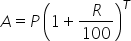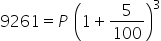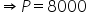Maths-
General
Easy

Question

# What sum of money will amount to  Rs9261 in 3years at 5% per annum compound interest?Hint:

## The correct answer is: 8000 Rupees

### Complete step by step solution:Let the principal amount = PHere we have R = 5% , T = 3 years and total amount A = 9261 Rupees.We know that…(i)On substituting the known values in (i), we getRupees.Hence the principal amount P = 8000 Rupees.#### With Turito Foundation.#### Get an Expert Advice From Turito.